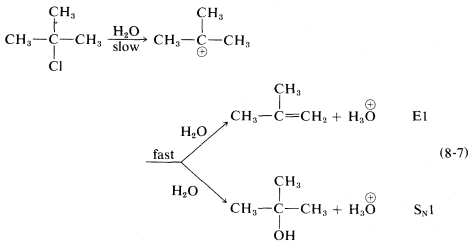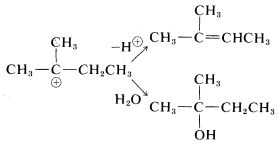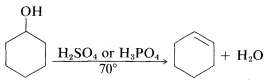# 8.10: The E1 Reaction

$$\newcommand{\vecs}{\overset { \rightharpoonup} {\mathbf{#1}} }$$ $$\newcommand{\vecd}{\overset{-\!-\!\rightharpoonup}{\vphantom{a}\smash {#1}}}$$$$\newcommand{\id}{\mathrm{id}}$$ $$\newcommand{\Span}{\mathrm{span}}$$ $$\newcommand{\kernel}{\mathrm{null}\,}$$ $$\newcommand{\range}{\mathrm{range}\,}$$ $$\newcommand{\RealPart}{\mathrm{Re}}$$ $$\newcommand{\ImaginaryPart}{\mathrm{Im}}$$ $$\newcommand{\Argument}{\mathrm{Arg}}$$ $$\newcommand{\norm}{\| #1 \|}$$ $$\newcommand{\inner}{\langle #1, #2 \rangle}$$ $$\newcommand{\Span}{\mathrm{span}}$$ $$\newcommand{\id}{\mathrm{id}}$$ $$\newcommand{\Span}{\mathrm{span}}$$ $$\newcommand{\kernel}{\mathrm{null}\,}$$ $$\newcommand{\range}{\mathrm{range}\,}$$ $$\newcommand{\RealPart}{\mathrm{Re}}$$ $$\newcommand{\ImaginaryPart}{\mathrm{Im}}$$ $$\newcommand{\Argument}{\mathrm{Arg}}$$ $$\newcommand{\norm}{\| #1 \|}$$ $$\newcommand{\inner}{\langle #1, #2 \rangle}$$ $$\newcommand{\Span}{\mathrm{span}}$$$$\newcommand{\AA}{\unicode[.8,0]{x212B}}$$

## Scope and Mechanism

Many secondary and tertiary halides undergo $$\text{E}1$$ elimination in competition with the $$\text{S}_\text{N}1$$ reaction in neutral or acidic solutions. For example, when tert-butyl chloride solvolyzes in $$80\%$$ aqueous ethanol at $$25^\text{o}$$, it gives $$83\%$$ tert-butyl alcohol by substitution and $$17\%$$ 2-methylpropene by elimination:The ratio of substitution and elimination remains constant throughout the reaction, which means that each process has the kinetic order with respect to the concentration of tert-butyl halide. The $$\text{S}_\text{N}1$$ and $$\text{E}1$$ reactions have a common rate-determining step, namely, slow ionization of the halide. The solvent then has the choice of attacking the intermediate carbocation at the positive carbon to effect substitution, or at a $$\beta$$ hydrogen to effect elimination:Factors influencing the $$\text{E}1$$ reactions are expected to be similar to those for the $$\text{S}_\text{N}1$$ reactions. An ionizing solvent is necessary, and for easy reaction the $$RX$$ compound must have a good leaving group and form a relatively stable $$R^\oplus$$ cation. Therefore the $$\text{E}1$$ orders of reaction rates are $$X = I$$ $$>$$ $$Br$$ $$>$$ $$Cl$$ $$>$$ $$F$$ and tertiary $$R$$ $$>$$ secondary $$R$$ $$>$$ primary $$R$$.

With halides such as 2-chloro-2-methylbutane, which can give different alkenes depending on the direction of elimination, the $$\text{E}1$$ reaction is like the $$\text{E}2$$ reaction in tending to favor the most stable or highly substituted alkene:## Rearrangement of Carbon Cations

Another feature of $$\text{E}1$$ reactions (and also of $$\text{S}_\text{N}1$$ reactions) is the tendency of the initially formed carbocation to rearrange, especially if a more stable carbocation is formed thereby. For example, the very slow $$\text{S}_\text{N}1$$ solvolysis of neopentyl iodide in methanoic acid leads predominantly to 2-methyl-2-butene:In this reaction, ionization results in migration of a methyl group with its bonding pair of electrons from the $$\beta$$ to the $$\alpha$$ carbon, thereby transforming an unstable primary carbocation to a relatively stable tertiary carbocation. Elimination of a proton completes the reaction.

Rearrangements involving shifts of hydrogen (as $$H:^\ominus$$) occur with comparable ease if a more stable carbocation can be formed thereby:Rearrangements of carbocations are among the fastest organic reactions known and must be reckoned with as a possibility whenever carbocation intermediates are involved.

## Acid-Catalyzed Elimination Reactions

Alcohols and ethers rarely undergo substitution or elimination unless strong acid is present. As we noted in Section 8-7D the acid is necessary to convert a relatively poor leaving group ($$HO^\ominus$$, $$CH_3O^\ominus$$) into a relatively good one ($$H_2O$$, $$CH_3OH$$). Thus the dehydration of alcohols to alkenes is an acid-catalyzed reaction requiring strong acids such as sulfuric or phosphoric acid:These are synthetically useful reactions for the preparation of alkenes when the alkene is less available than the alcohol. They can occur by either the $$\text{E}1$$ or $$\text{E}2$$ mechanism depending on the alcohol, the acid catalyst, the solvent, and the temperature.